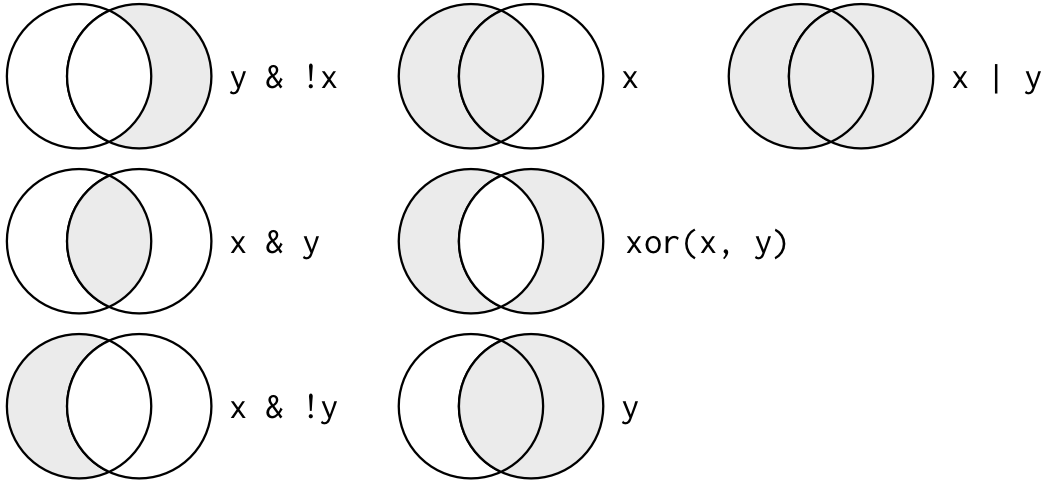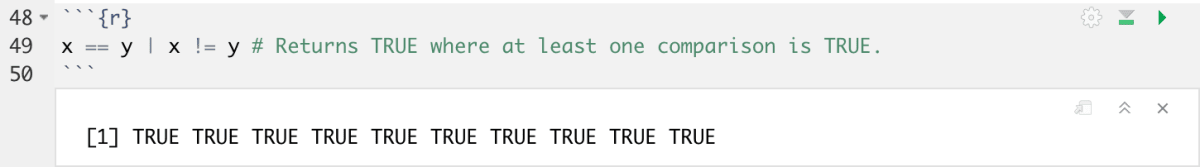Skip to content

# Operators

Operators are special symbols that carry out some sort of computation. They can be applied to scalar quantities, vectors and matrices. Here are some commonly used operators:

### Arithmetic:

 Operator Notation Example addition + x+y multiplication * x*y exponentiation ** x**y

### Logical:

 Operator Notation Example equal == x == y not equal != x != y greater than or equal to >= x >= y and & x>y & x>z or | x>y | x>zFigure: Complete set of boolean operations. `x` is the left-hand circle, `y` is the right-hand circle, and the shaded region show which parts each operator selects.

### Example:

In this example, let’s go through a script in which the operators are used in combination. We are creating two vectors, x (1,2,3…10) and y (10,9,8…1). We would like to check two conditions using the operators:

AND: `&` If x is greater than y, and x is greater than 5, return TRUE. Both conditions must be met to return `TRUE`.

```x <- 1:10
y <- 10:1
x > y & x > 5 # Returns TRUE where both comparisons return TRUE.```OR: `|` We are checking a new condition now: If x is equal to y or x is not equal to y, return true. Either condition may be met to return `TRUE`.

`x == y | x != y # Returns TRUE where at least one comparison is TRUE.`